Disclosure: This post may contain affiliate links, meaning when you click the links and make a purchase, we receive a commission.

# Creating Email Addresses from a Single Column with Flash Fill, TEXT Formulas & Commentator’s Text Formula Suggestions

## Using Flash Fill

This is a question that is often asked namely, how to extract data from one column into another format or more than one column.

We are going to see how to do this using the Flash Fill feature first. Flash Fill is a new feature that was added to Microsoft Excel 2013 and later versions.

Read More: Splitting Text in Excel Using Flash Fill

In the example below, we have a list of names and surnames in Column A, Flash Fill always needs a source column, in order to work, which in this case is Column A, which contains the staff names. Flash Fill is often used in examples to generate email addresses or other types of formulaic repetitive data. Text formulas are also used extensively for the email generation question. There are a variety of text formulas that one can use, from the more complex to the more simple. In this article we have a flash fill email generation example, a text formula email generation example and we’ve updated with commentator’s text formula suggestions.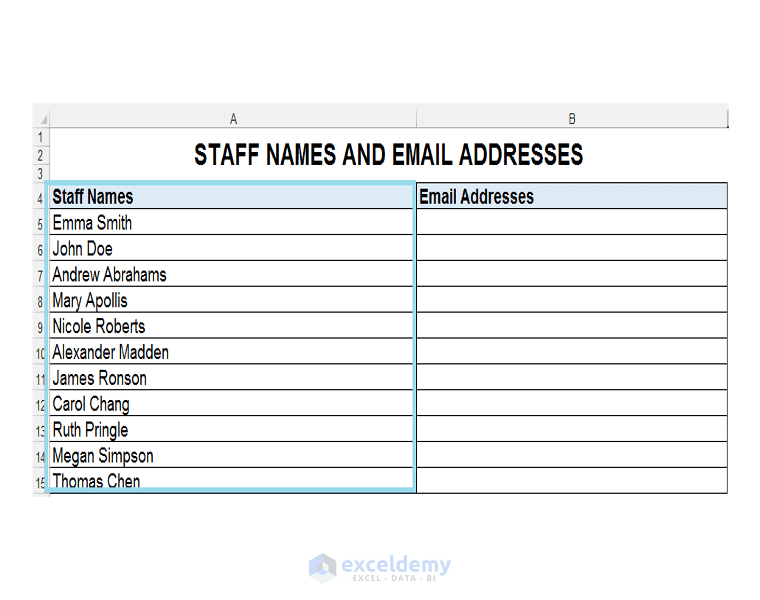1) We would like to populate Column B, with the email addresses of the staff members. The way the email addresses are created by the company’s internal IT, is that the name and the surname of the staff member is combined with the company’s domain name, to produce the email address.

2) In Cell B5, we have to give Flash Fill, an example of what we want first and Flash Fill will then use this as a template in order to populate the rest of the column. So we enter the text:

EmmaSmith@ourcompany.com

We then press CTRL-ENTER.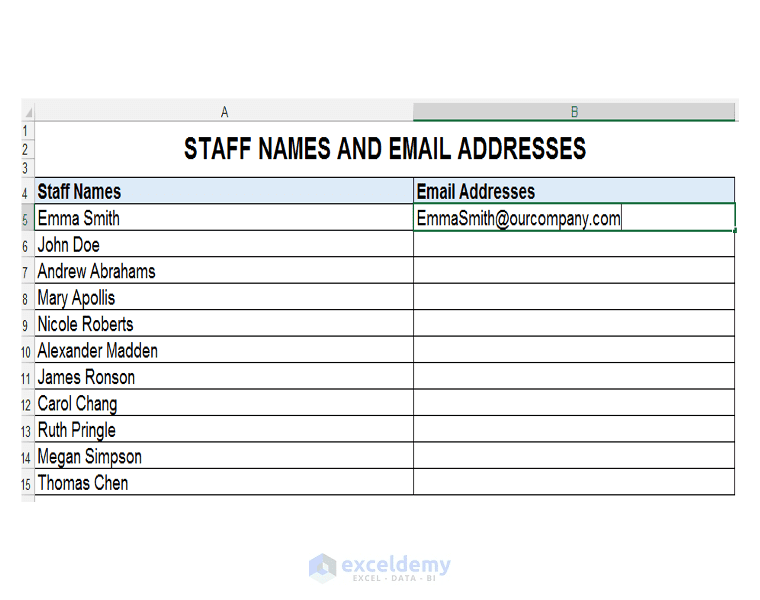3) After one presses CTRL-ENTER, Excel changes the text to a hyperlink automatically.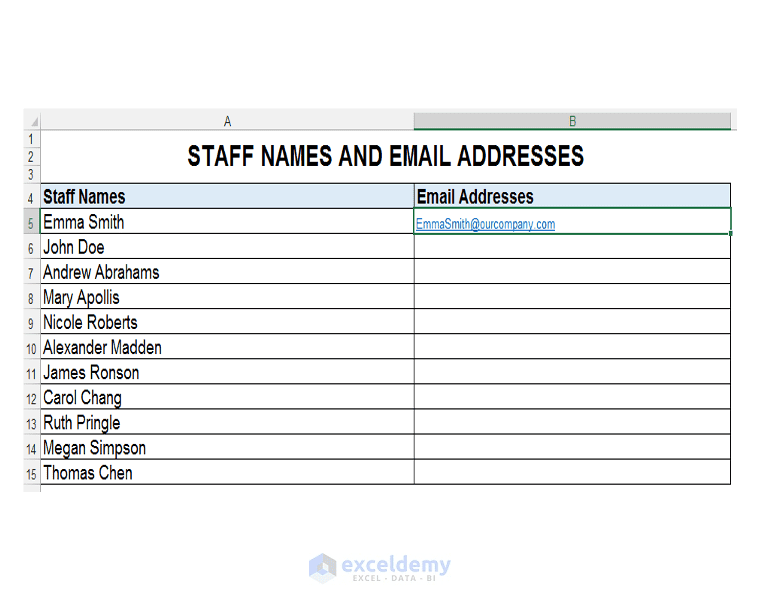4) We then have to highlight the entire range B5:B15 that we want to populate with email addresses, using Flash Fill and either press CTRL-E, which is the shortcut key for Flash Fill or with the range highlighted go to the Data tab, and click on Flash Fill as shown.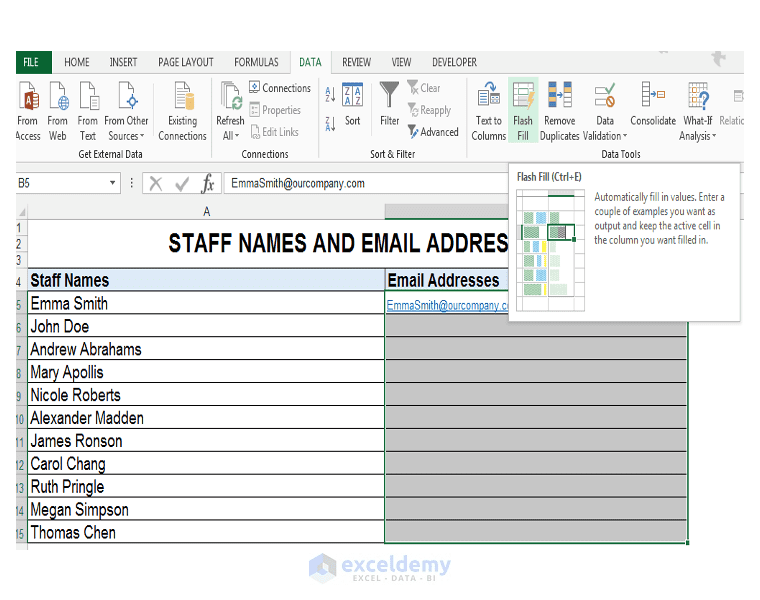5) The rest of the range is filled with the email addresses needed.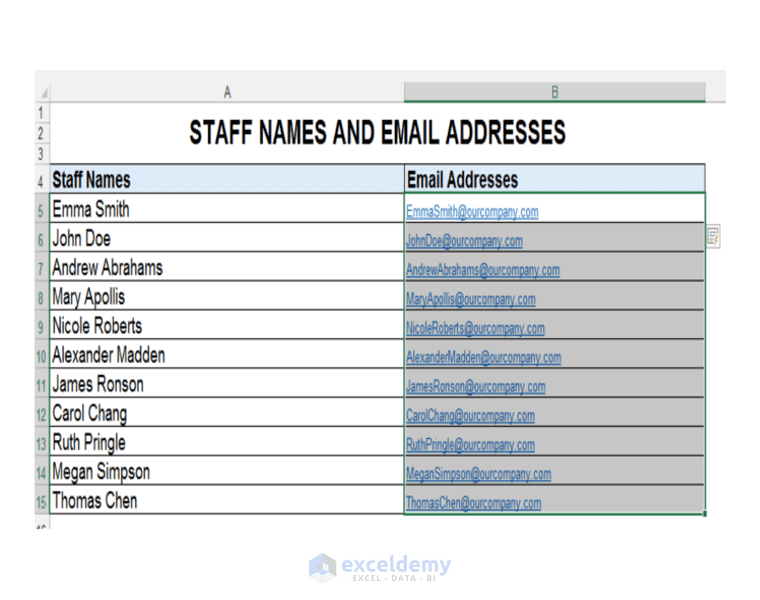## Using a TEXT Formula

All is not lost if you have an earlier version of Microsoft Excel, since we can do the same thing with TEXT formulas. Using TEXT formulas is slightly more complicated however, in this particular case.

1) Firstly, we have to look at the text in Column A, in order to figure out which TEXT functions to use in our complex formula.2) We see that we have a first name, followed by a space and then the last name.

3) We are going to build this formula up from scratch.

The first thing, we do is use the SEARCH function as shown to find the space, and in Cell B5, we enter:

=SEARCH (” “, A5, 1)

Press CTRL-ENTER.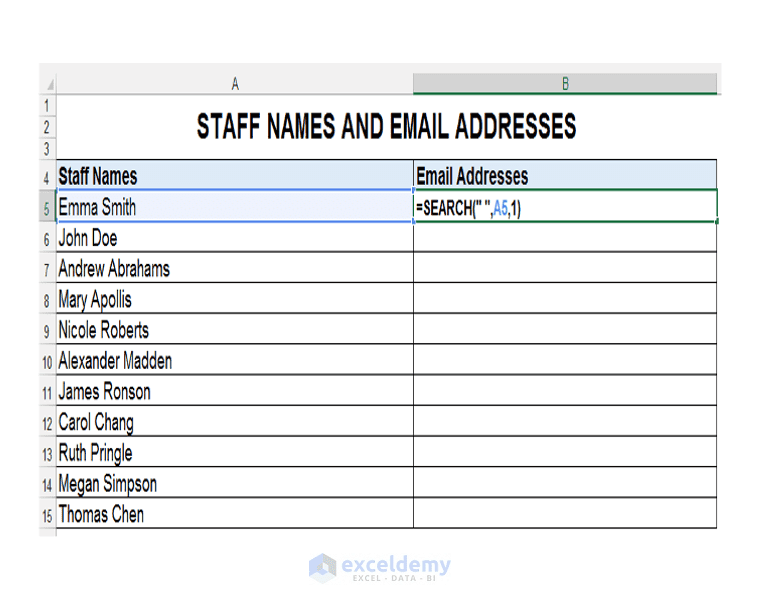4) After pressing CTRL-ENTER we get 5, in other words in the case of Emma Smith, the space is the fifth character. Double clicking and sending the formula down, shows us where the space occurs for all the other staff members. For Nicole Roberts, the space is the seventh character in the text string as shown.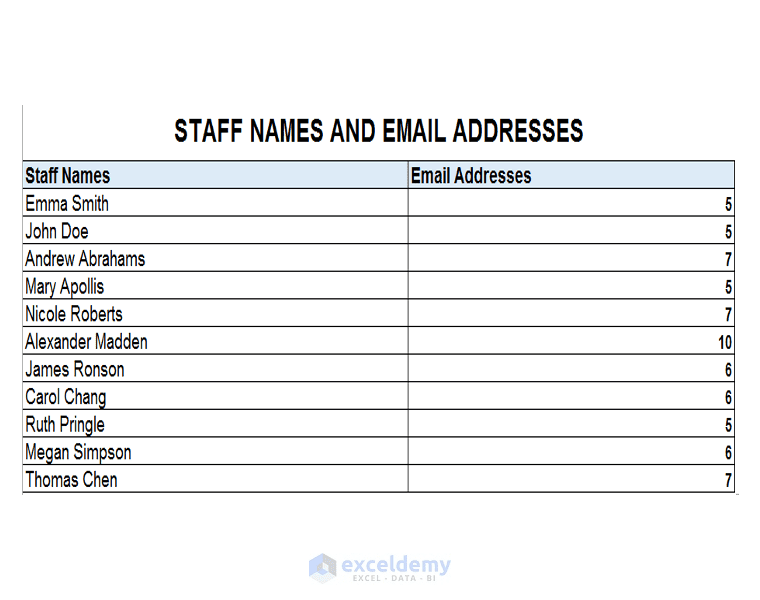5) We can now use the LEFT function to extract the first name, since based on where the space is, we can extract all the characters, left of the space to get the first name.

So, we need to give the LEFT function the input text string, and then the numbers of characters in the text string from the left that we’d like to extract.

So, we select Cell A5, as the text string, and then to find the number of characters to extract from the left to get the first name only, we use the SEARCH function and we subtract 1 to get:

=LEFT (A5, SEARCH (” “, A5, 1) -1)

We subtract 1 because we do not want to include the space.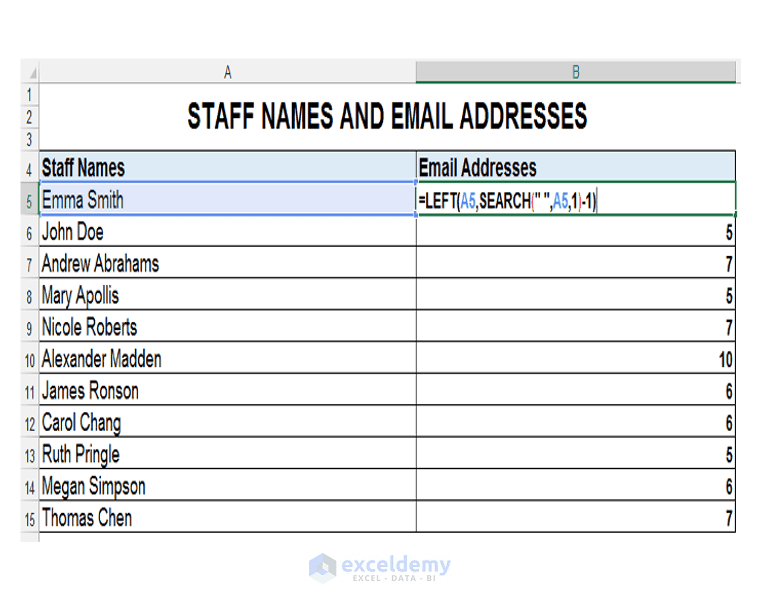6) Once we press CTRL-ENTER and double click the formula and send down. We get all the first names extracted as shown.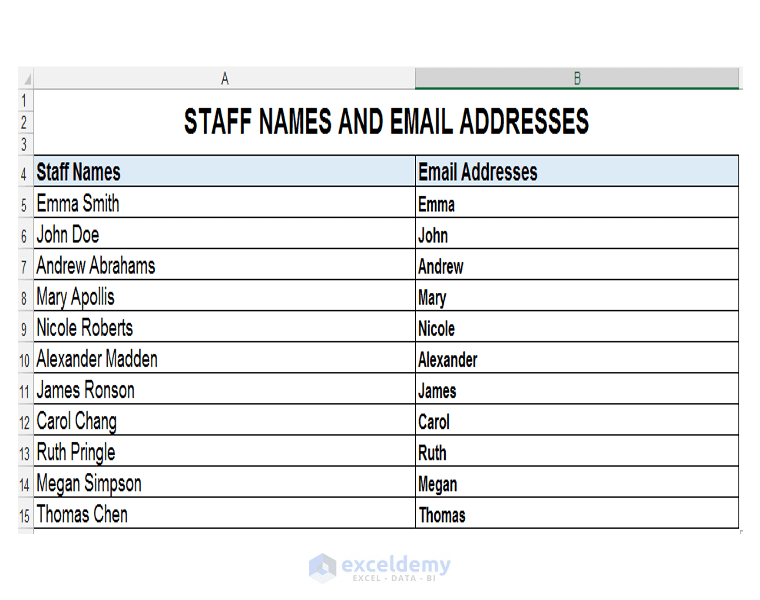7) We can now copy the formula by pressing CTRL-C twice, in order to open the Clipboard.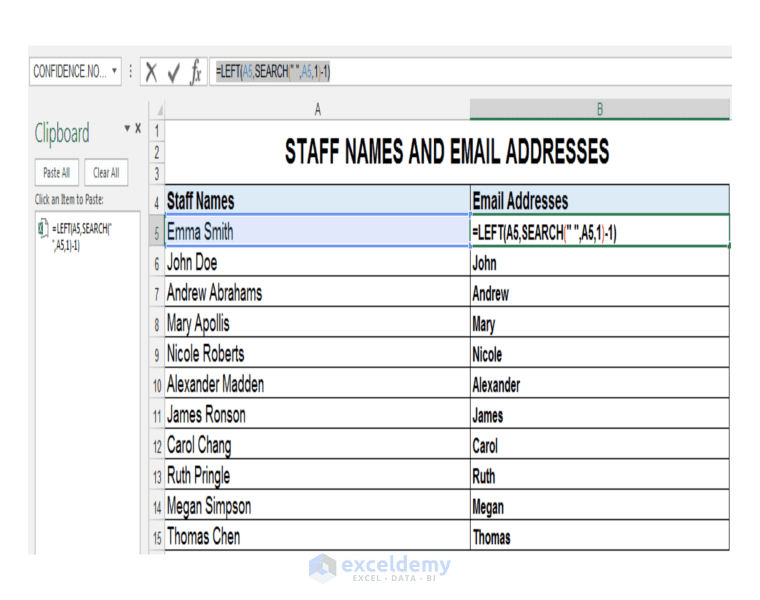8) We now have the formula stored for later use. So we can clear Column B and work on extracting the surname.

9) We know where the space is, and since we used the LEFT function to extract the first name, we could use the RIGHT function to extract the surname. So, let’s start building the formula for this process of extracting the surname.

10) We need to tell the RIGHT function the number of characters to extract from the right. We know the position of the space. Thus, we can use the LEN function to tell us the total amount of characters in the text string and then subtract the leftover from the SEARCH function, used to tell us initially where the space is.

We use the following formula:

=LEN (A5) – SEARCH (” “, A5, 1))

This gives us the leftover characters after the space.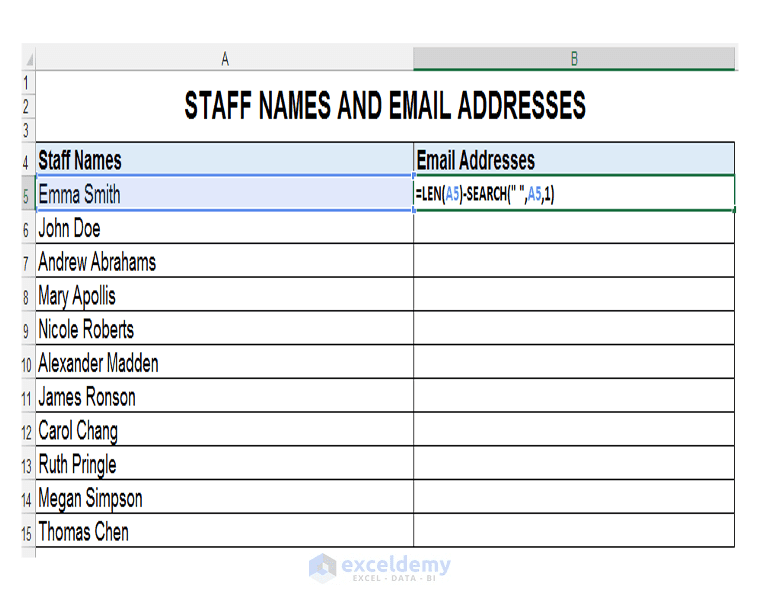11) We then can use the RIGHT function in conjunction with the above formula to extract the surname, since we now know the number of characters from the right to extract to get to the space.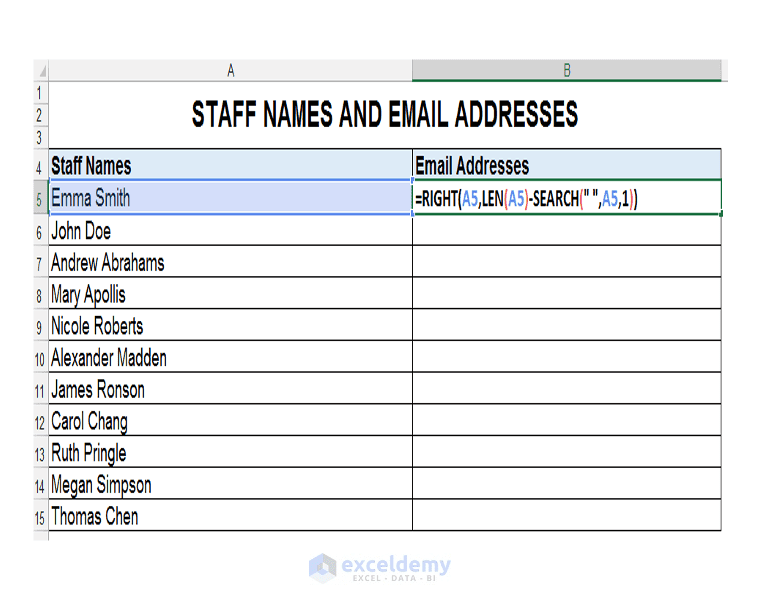So, we type the following formula:

=RIGHT (A5, LEN (A5) – SEARCH (” “, A5, 1))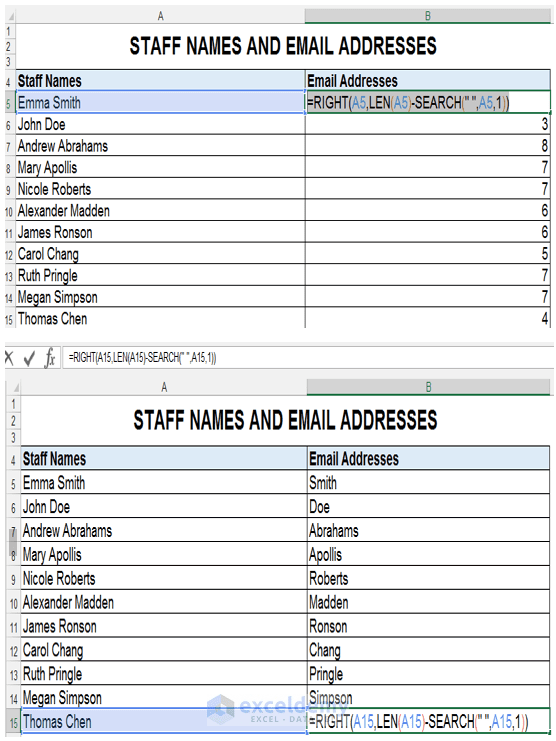13) Copy the formula and then clear the contents of the cell.

14) We can now put everything together in one big formula, since we’ve broken each step down in order to show the logic, and copied each section to the Clipboard.

Read More: Split one column into multiple columns in Excel [Text to Columns]

So, after clearing the column, in Cell B5, we type the formula:

= LEFT (A5, SEARCH (” “, A5, 1) – 1)&RIGHT (A5, LEN(A5) – SEARCH(” “,A5,1))&”@mycompany.com”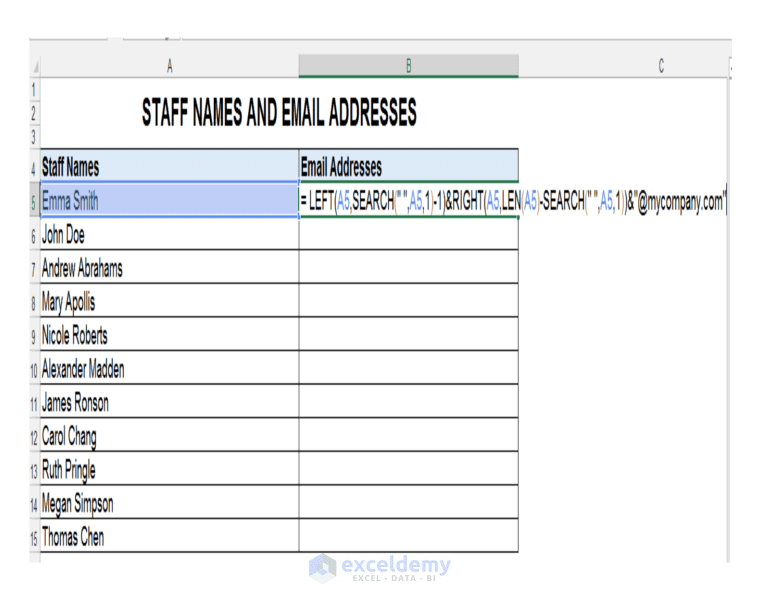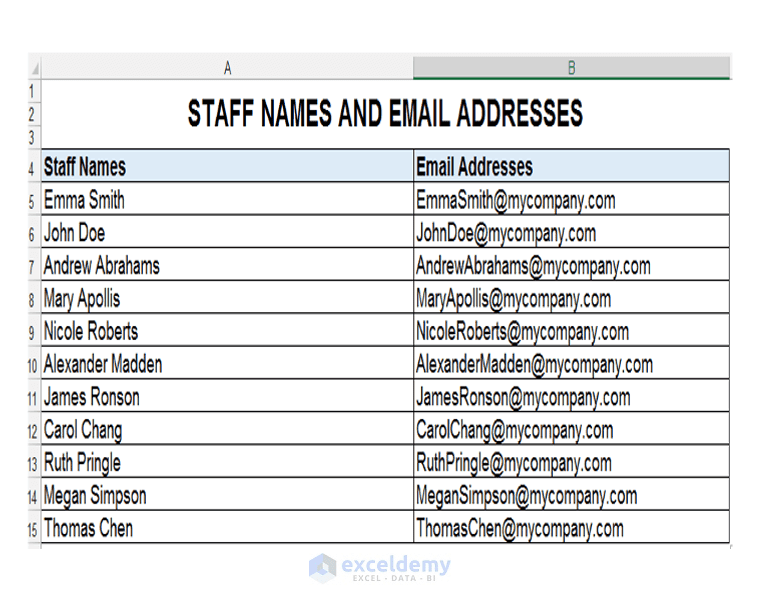15) We double click and send down, in order to populate the rest of the column with the email addresses.

Excellent Commentator Suggestions to achieve the same effect:

Rahul Singh contributed the following formula to achieve the same effect, using a much less complex formula:
So in cell B5 type the following formula:

=CONCATENATE(SUBSTITUTE(A5,” “, “”),”@mycompany.com”)

Karthik contributed the following formula to achieve the same effect as the initial text formula in the post, it is also much less complex:

=SUBSTITUTE(A5,” “,””)&”@mycompany.com”

## Working File

Flash-Fill-TEXT-Function-Excel

## Conclusion

Flash Fill is a handy new feature in Microsoft Excel 2013 and later. It is an enhanced AutoFill and can be quite time saving. However, for those using earlier versions of Excel and in certain cases where Flash Fill does not work, TEXT formulas come to the rescue. While they are more complex to create, there is no example too complicated for them to tackle. Please feel free to comment and tell us if you prefer using Flash Fill or TEXT formulas to extract/format data and why.

## Related Articles

Taryn is a Microsoft Certified Professional, who has used Office Applications such as Excel and Access extensively, in her interdisciplinary academic career and work experience. She has a background in biochemistry, Geographical Information Systems (GIS) and biofuels. She enjoys showcasing the functionality of Excel in various disciplines.

1. ReplyKarthik Mar 23, 2017 at 10:41 AM

=SUBSTITUTE(A5,” “,””)&”mycompany.com”

It gives same answer but less complicated…:)

• Reply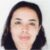Mar 23, 2017 at 8:25 PM

I’ve updated the article with your suggestion just added the @ symbol, as well as commentator Rahul Singh’s. Thanks for the formula suggestion and please keep contributing. 🙂

2. ReplyRAHUL SINGH Mar 23, 2017 at 12:09 PM

Hi Taryn N!!!!!

Thanks for sharing such an awesome Excel Function.

I would like to suggest some modification. In the second sheet of the above file in which you have you used the TEXT function is quite complex and difficult to understand for determining the email address.

We can use the below formula instead:

=CONCATENATE(SUBSTITUTE(A5,” “,””),”@mycompany.com”)

Put the above formula in cell B5 in the “TextFormula” sheet and drag it down till the last field.

Hope it helps!!!!;););):)

• ReplyMar 23, 2017 at 8:05 PM

Thank you, I am going to update the article with your formula suggestion. I know the text formula is complicated – it was mainly for demonstration purposes of how LEN, RIGHT, LEFT and other text functions can work together to deliver results 🙂

3. ReplyStephen Sep 19, 2017 at 2:58 AM

Many thanks for the fantastic learning tips keep it up

• ReplyDec 16, 2017 at 10:47 PM

🙂 Thank you so much 🙂